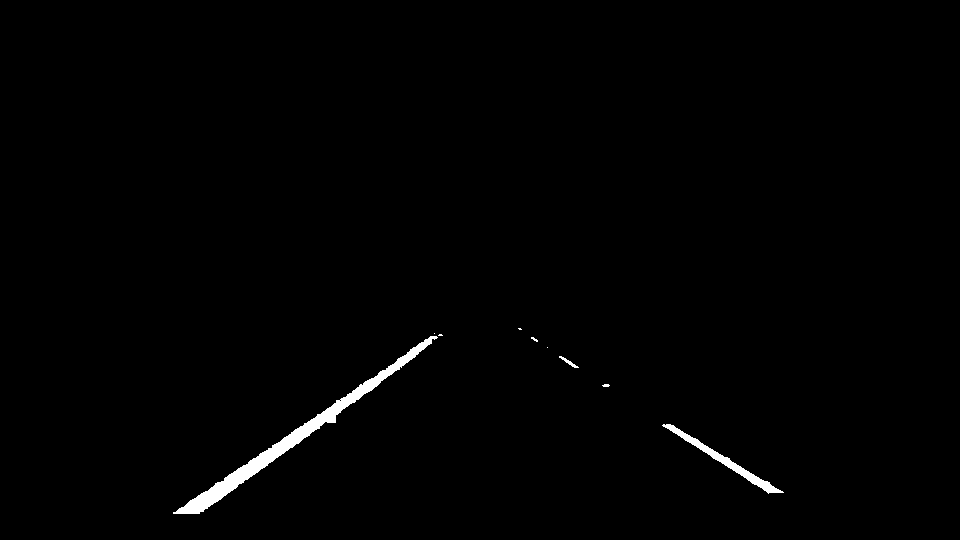# 选择图像的“感兴趣区域”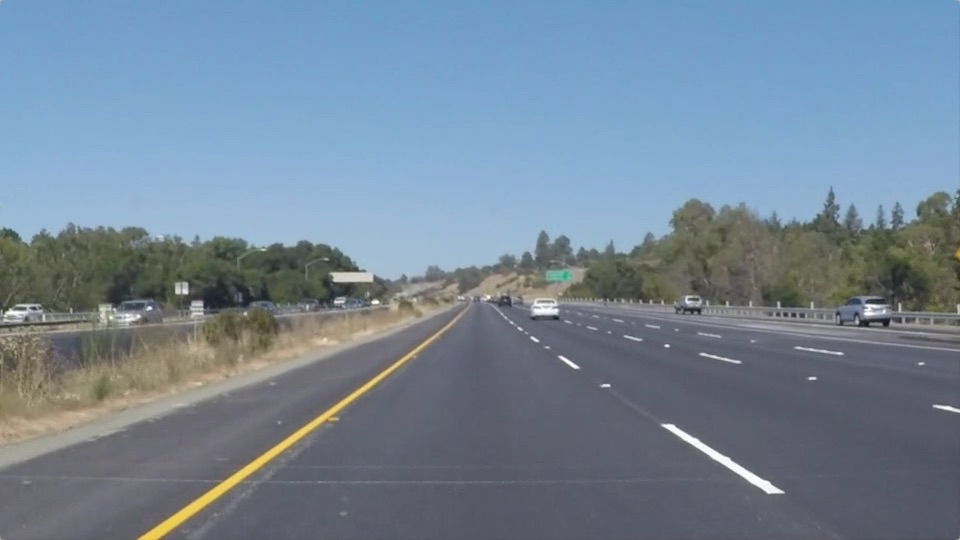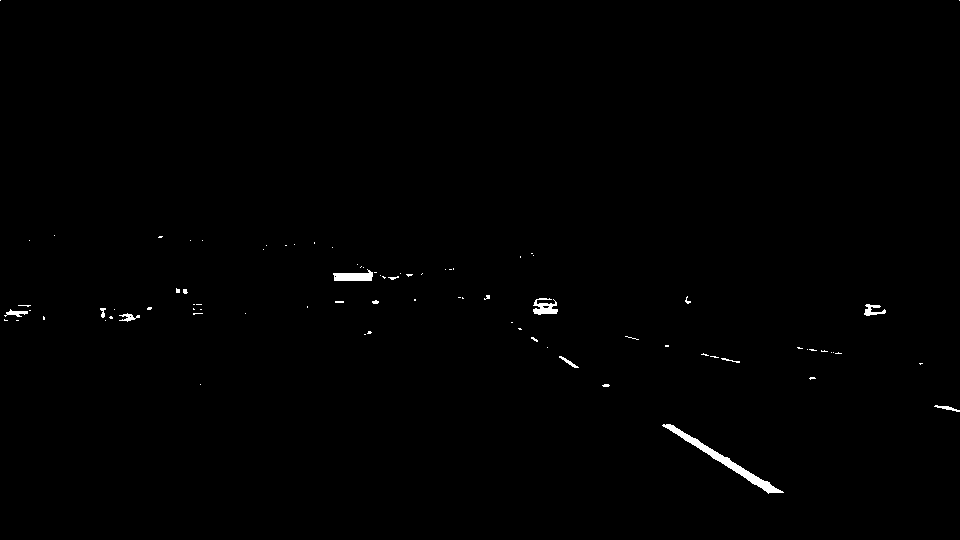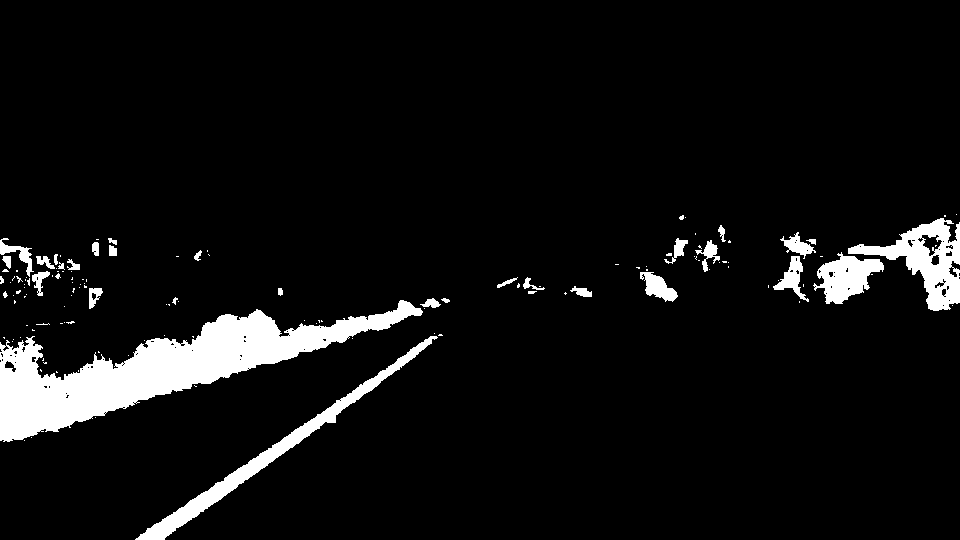import cv2
mixed = cv2.bitwise_or(white, yellow)
cv2.imshow("mixed", mixed)
cv2.waitKey()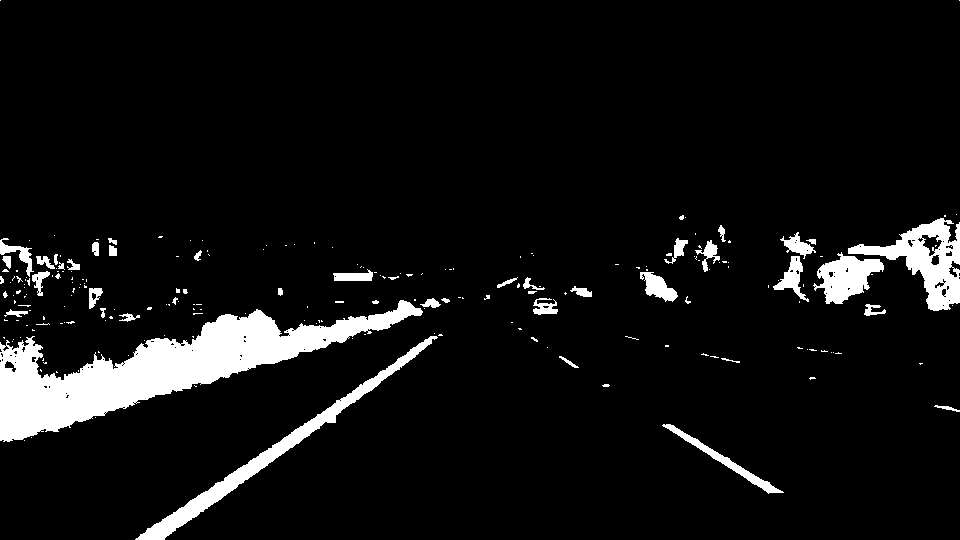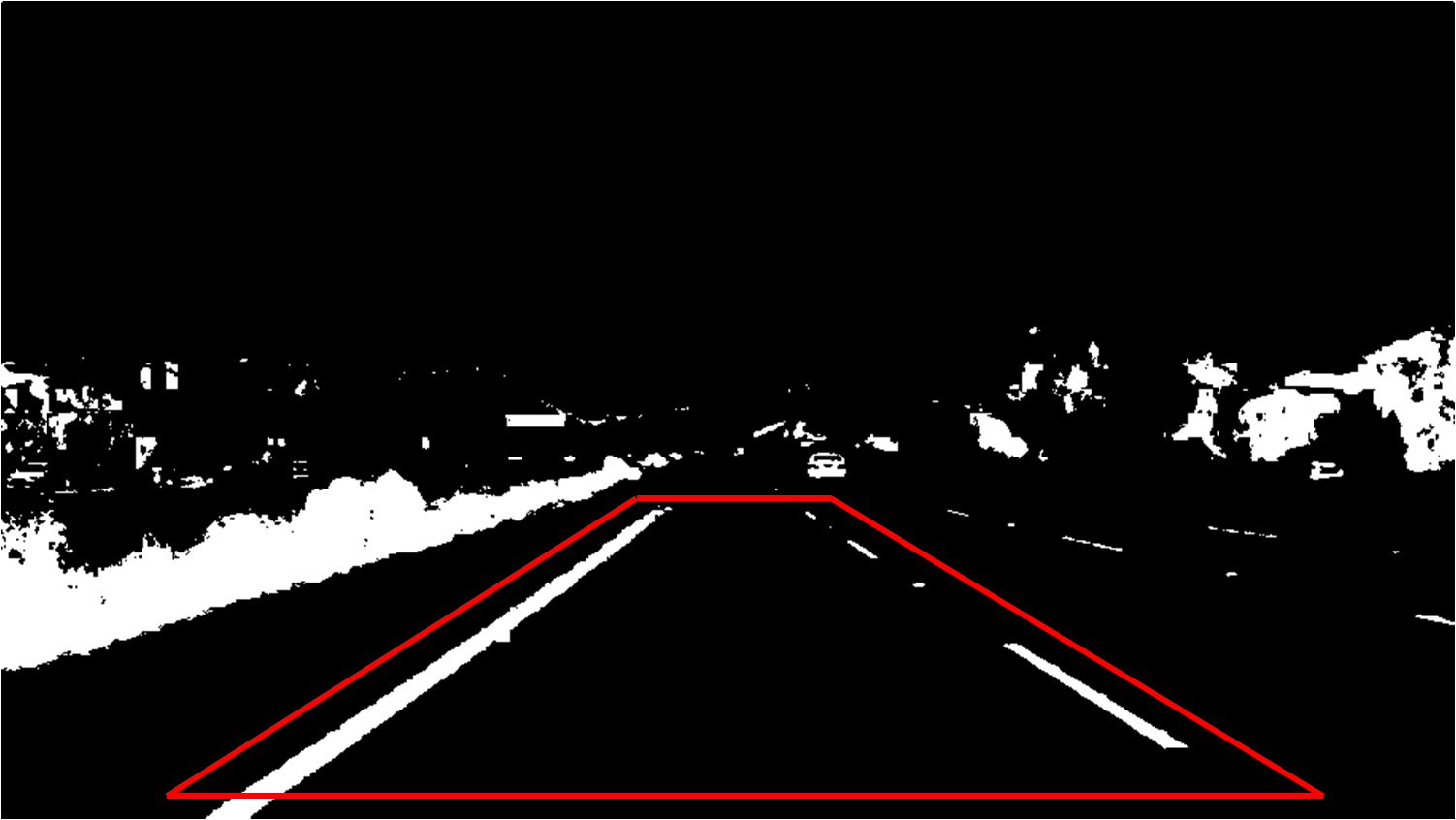# 获取原始图像的行和列
row, col = img.shape[:2]
# 定义多边形的顶点
bottom_left = [col * 0.05, row]
top_left = [col * 0.45, row * 0.6]
top_right = [col * 0.55, row * 0.6]
bottom_right = [col * 0.95, row]
# 使用顶点定义多边形
vertices = np.array([bottom_left, top_left, top_right, bottom_right], dtype=np.int32)

roi_mask = np.zeros((row, col), dtype=np.uint8)
cv2.waitKey()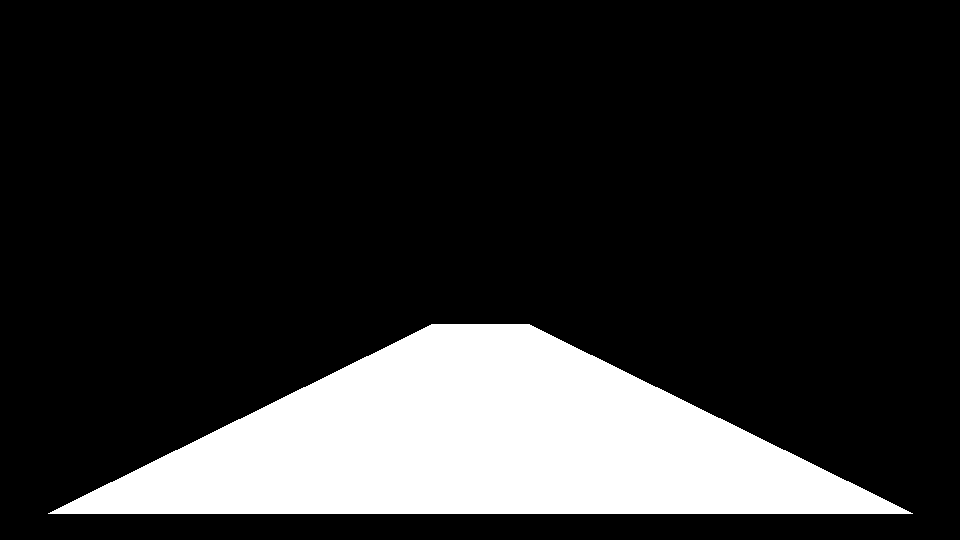roi = cv2.bitwise_and(img, img, mask=roi_mask)
cv2.imwrite("final_roi.png", roi)
cv2.waitKey()